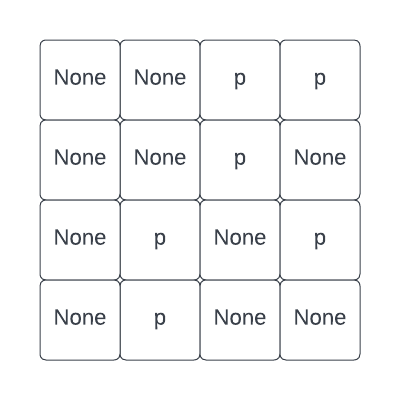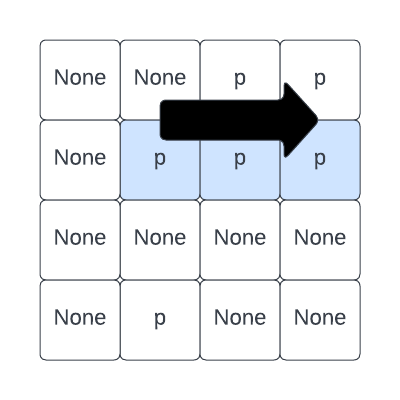Computer Science

# More spatial Logic

We want to write a function that returns true if there are three consecutive puppies any direction (left, right, up, down, diagonal).Let’s imagine we are checking a grid, moving from left to right:We do not need to also check from right to left! Any group of consecutive puppies we find going right would also be found going left — but we don’t need to check both. The same applies to lookup up/down and diagonally.

This limits our searching a bit. We only need to check four directions:

• going right
• going down
• going down and to the left
• going down and to the right

## Using guards

Let’s re-use the is_puppy() function with guards. We wrote this previously.

def is_puppy(grid, row, column):
num_rows = len(grid)
num_columns = len(grid)

# check if the row is off the grid
if row < 0 or row >= num_rows:
return False

# check if the column is off the grid
if column < 0 or column >= num_columns:
return False

if grid[row][column] == 'p':
return True

return False

This will ensure we never check out of bounds on the grid.

## Checking for consecutive puppies

Now we can write functions checking for consecutive puppies without worrying about the edges of the grid. First, a function to look to the right:

def has_three_right(grid, row, column):
for c in range(column, column + 3):
if not is_puppy(grid, row, c):
return False

return True

We look at three columns in a row, starting with our current column. And if there is not a puppy in any of these cells, we can immediately return False. If we get through all three columns and find a puppy everywhere, we return True.

Similarly, we can write a function to check down:

def has_three_down(grid, row, column):
for r in range(row, row + 3):
if not is_puppy(grid, r, column):
return False

return True

a function to check down and to the left:

def has_three_down_left(grid, row, column):
for r, c in zip(range(row, row + 3), range(column, column - 3, -1)):
if not is_puppy(grid, r, c):
return False

return True

and a function to check down and to the right:

def has_three_down_right(grid, row, column):
for r, c in zip(range(row, row + 3), range(column, column + 3)):
if not is_puppy(grid, r, c):
return False

return True

Notice that for these last two we use zip! This lets us look diagonally. For example, to go up and to the right, we are zipping the lists [row, row + 1, row +2] and [column, column + 1, column + 2] to get a list of tuples: [(row, column), (row + 1, column + 1), (row + 2, column + 2)].

In addition, in all of these functions we use r for ranges counting rows and c for ranges counting columns, so we have to be sure to use r and/or c when checking for puppies.

## Checking for three in a row

Now we can write the primary function:

def has_three_in_a_row(grid, value='X'):
num_rows = len(grid)
num_columns = len(grid)
for row in range(num_rows):
for column in range(num_columns):
if has_three_right(grid, row, column)
or has_three_down(grid, row, column) \
or has_three_down_left(grid, row, column) \
or has_three_down_right(grid, row, column):
return True
return False

We are using \ to continue the if statement on multiple lines since it is really long

## Running the code

We can run this code with:

park1 = [
[None, None, 'p', 'p'],
[None, 'p', 'p', 'p'],
[None, None, None, None],
[None, 'p', None, None]
]

park2 = [
[None, None, 'p', 'p'],
[None, None, 'p', None],
[None, 'p', None, 'p'],
[None, 'p', None, None]
]

park3 = [
[None, None, None],
[None, None, None],
[None, None, None]
]

print(has_three_in_a_row(park1))
print(has_three_in_a_row(park2))
print(has_three_in_a_row(park3))

We get:

True
True
False